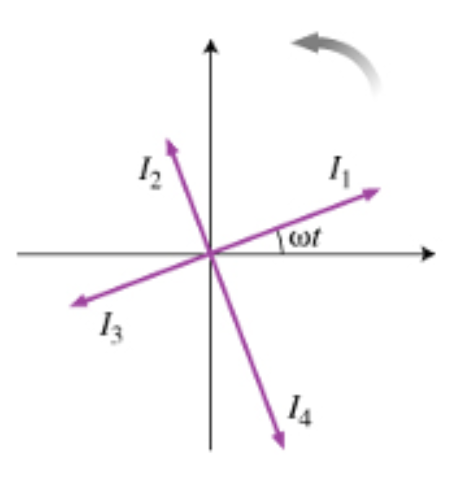# Problem: Let us assume that a certain quantity I(t) changes over time as I(t) = I0cos(ωt). A phasor is a vector whose length represents the amplitude I0 (see the diagram (Figure 1) ). This vector is assumed to rotate counterclockwise with angular frequency ω, that way, the horizontal component of the vector represents the actual value i(t) at any given moment. In this problem, you will answer some basic questions about phasors and prepare to use them in the analysis of various AC circuits. At the moment shown in the diagram, which of the following statements is true?A) I2 lags I3 by πB) I3 lags I2 by 2πC) I2 lags I3 by π/2D) I3 lags I2 by π/2

###### FREE Expert Solution

I3 is ahead of I2 by π/2 (or 90°)

83% (351 ratings)###### Problem Details

Let us assume that a certain quantity I(t) changes over time as I(t) = I0cos(ωt). A phasor is a vector whose length represents the amplitude I0 (see the diagram (Figure 1) ). This vector is assumed to rotate counterclockwise with angular frequency ω, that way, the horizontal component of the vector represents the actual value i(t) at any given moment. In this problem, you will answer some basic questions about phasors and prepare to use them in the analysis of various AC circuits.At the moment shown in the diagram, which of the following statements is true?

A) I2 lags I3 by π

B) I3 lags I2 by 2π

C) I2 lags I3 by π/2

D) I3 lags I2 by π/2